Publicité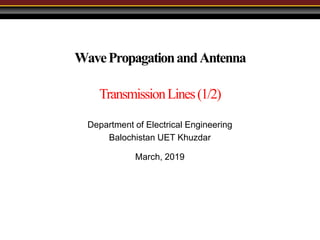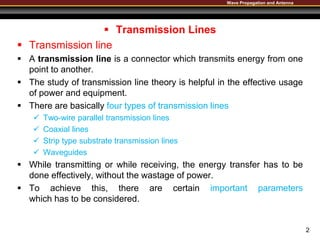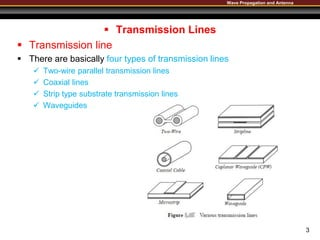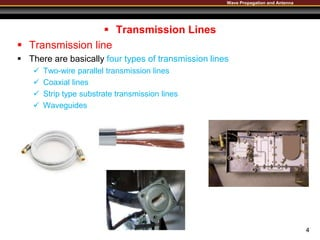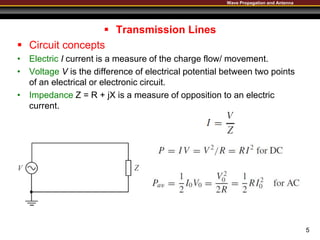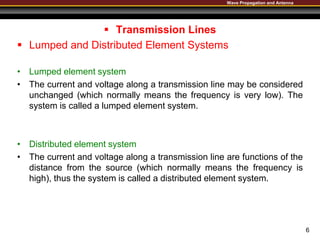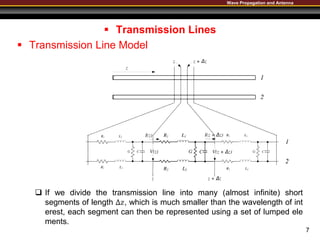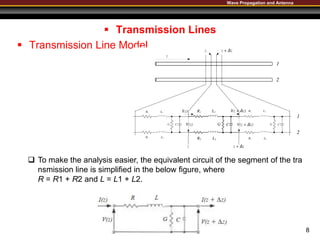1 sur 8
Publicité

### 02 Lecture Transmission Lines 02.pptx

1. WavePropagationandAntenna TransmissionLines(1/2) March, 2019 Department of Electrical Engineering Balochistan UET Khuzdar
2. Wave Propagation and Antenna  Transmission Lines  Transmission line  A transmission line is a connector which transmits energy from one point to another.  The study of transmission line theory is helpful in the effective usage of power and equipment.  There are basically four types of transmission lines  Two-wire parallel transmission lines  Coaxial lines  Strip type substrate transmission lines  Waveguides  While transmitting or while receiving, the energy transfer has to be done effectively, without the wastage of power.  To achieve this, there are certain important parameters which has to be considered. 2
3. Wave Propagation and Antenna  Transmission Lines  Transmission line  There are basically four types of transmission lines  Two-wire parallel transmission lines  Coaxial lines  Strip type substrate transmission lines  Waveguides 3
4. Wave Propagation and Antenna  Transmission Lines  Transmission line  There are basically four types of transmission lines  Two-wire parallel transmission lines  Coaxial lines  Strip type substrate transmission lines  Waveguides 4
5. Wave Propagation and Antenna  Transmission Lines  Circuit concepts • Electric I current is a measure of the charge flow/ movement. • Voltage V is the difference of electrical potential between two points of an electrical or electronic circuit. • Impedance Z = R + jX is a measure of opposition to an electric current. 5
6. Wave Propagation and Antenna  Transmission Lines  Lumped and Distributed Element Systems • Lumped element system • The current and voltage along a transmission line may be considered unchanged (which normally means the frequency is very low). The system is called a lumped element system. • Distributed element system • The current and voltage along a transmission line are functions of the distance from the source (which normally means the frequency is high), thus the system is called a distributed element system. 6
7. Wave Propagation and Antenna  Transmission Lines  Transmission Line Model 7  If we divide the transmission line into many (almost infinite) short segments of length ∆𝑧, which is much smaller than the wavelength of int erest, each segment can then be represented using a set of lumped ele ments.
8. Wave Propagation and Antenna  Transmission Lines  Transmission Line Model 8  To make the analysis easier, the equivalent circuit of the segment of the tra nsmission line is simplified in the below figure, where R = R1 + R2 and L = L1 + L2.

### Notes de l'éditeur

1. Resistance, capacitance, inductance, conductance
2. Resistance, capacitance, inductance, conductance
3. Resistance, capacitance, inductance, conductance
Publicité